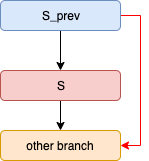# Matryoshka:Fuzzing Deeply Nested Branches

### 0x00 Questions### 0x01 The ways

1.1 分支条件的控制流依赖

\preccurlyeq 表示。传递性可以描述为

\text{if}\ a \preccurlyeq b, b \preccurlyeq c ,\text{then}\ a \preccurlyeq c

Prior(s) = IPrior(b) \cup IPrior(IPrior(b))\cdots

Intraprocedural immediate prior conditional statement：（ r of s

• rs在同一个函数内

• s 不是 rpost-dominator (post-dominator ：如果 rexit -point的所有路径都经过 s )

Interprocedural immediate prior conditional statementr of s

• rs 不在同一个函数内
• s 执行的时候， f_r 的返回地址在当前的栈中。
• r_cf_r 执行过的最后一个调用， r_c 不是 rpost-dominators

if(x==1){
exit();
}

if(y==2){//target
//...
}


1.2 分支条件的数据流依赖

b(s) 表示流到分支条件 s 上的input bytes集合， s 表示一个或者多个分支条件。当inputs 处可达，为了进入 s 代表的分支，需要进一步修改input, 在修改之后， Prior(s) 里面路径条件选择不变的情况下， s 还是reachable，从直觉上来说，为了尽可能小的影响 Prior(s) 里面的路径条件，需要尽可能的避免修改 b(Prior(s))input bytes,但是这样做有可能带来负反馈，例如F2中 b(Prior(s_{l6}))=\{x,y,z\} ,这将直接导致根本无法进入L6所代表的分支。

1.3 求解约束

1. Prioritize reachability
2. Prioritize satisfiability
3. Joint optimization for both reachability and satisfiability

1.3.1 Prioritize satisfiability

bracktrace过程从 s 向后按顺序fuzz EPrior(s) 里面的每个路径条件 r ,这个过程中需要避免修改流入$s$ 或者在 r 后面的effective prior conditional statementsinput bytes,bracktrace成功的标志是处理完所有*EPrior(s)*的路径条件。

1.3.3 Joint optimization for both reachability and satisfiability

• f_i(x)\leq 0,\forall i \in [1,n] 表示 EPrior(s) 中每一个路径条件的黑盒抽象函数，同样使用和Angora一样的转换表：

• g(x) = \sum_{i=0}^{n}R(f_i(x))\ ,R(x) \equiv 0 \vee x

其中 R(x) 相当于一个max函数， \vee 二元运算符表示取最大的那个操作数，并用 f_0(x) 表示 s 的抽象运算。观察这里转换表比Angora多了一个 \epsilon ,用于区别路径条件类型的最小正数。当 g(x)=0 的时候，必然有 \forall i\in[1,n],f_i(x)=0 , 在这里把 g(x)=0 作为预期最优解。（我觉得应该是 g(x)\le 0 ,如何保证每个 f_i(x)=0 这个事实？）。需要注意的是它这里要比Angora做的更好，因为Angora在计算偏导数的时候，并不能保证 sreachable，这样得到的结果没有意义，Angora面对这个问题，只是简单提到了通过改变单位分向量的值。这里Matryoshka可以强制保证previous分支选择，来保证计算过程。

同样这样也用F2中的例子来说明问题，让 [x,y]=[1,3] 为初始 input

g([x,y]) = R(x-2+\epsilon) +R(x+y-3+\epsilon) + R(1-y+\epsilon)

通过梯度下降，最终会得到一个solutiong([0,2])=0

1.4 隐式条件路径

L6计算显式的 EPrior(s_{l6})=\emptyset ,很显然L3应该是属于 EPrior(s_{l6}) 的，但是现有的方法是无法把它区分出来的，针对这种类似隐式的污点传递，通常的做法是如果路径条件被污染，则把该分支下的所有赋值操作都视为污点传递。这种方法文中提到，可能会导致over tainttaint explosion 。（但是我认为这样做理论上并没有问题，例如L4中n也被tainted,作者认为是useless，就算它是useless也不会影响其他的东西，考虑到因为这些污点导致更深的传递过程，也是合乎情理的，这属于一种indirect tainted,我猜测处理它的难点在于与之关联的input bytes不好做对应，这个过程需要手工的添加一些额外的taint rule

• 所有在r之前的条件路径

• 所有显式的effective prior conditional statements

• 所有已知的隐式的effective prior conditional statements

y=0;
z=input();
if(z){
y=1;
x=2;
}

if(y){
useless=1;
}

if(x==2){
if(a==19970131){//target conditional statement
//...
}
}


• L4:true;L8:true;L12:true
• L4:false;L8:false;L12:false

• L12不属于 EPrior(s) ，按照验证算法，这里是L13是unreachable的，所以L12属于隐式的effective prior conditional statement

• L8不属于 EPrior(s) ，按照验证算法，这里L13还是reachable的，所以L8不属于隐式的effective prior conditional statement

• L3不属于 EPrior(s) ，按照验证算法，这里是L13是reachable的，所以L3不属于隐式的effective prior conditional statement

1 Like